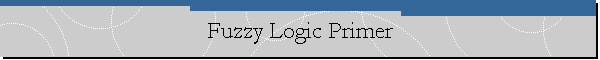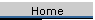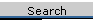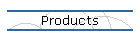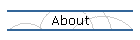Delphi CodeSmith Blog

# What is Fuzzy Logic?

Just what is Fuzzy Logic? Fuzzy Logic was actually invented by Lofti Zadeh way back in 1965. Despite its name, Fuzzy Logic is not the science of fuzzy thinking combined with fuzzy conclusions :-).  Fuzzy Logic is a method for modeling and reasoning about imprecise or approximate concepts. Traditional logic, where concepts must be true or false, zero or one, has trouble at the boundaries of a problem: Is the water hot or cold? It's neither and both, it is somewhat hot. Fuzzy logic concerns itself with the boundaries of a concept, things can be "somewhat true" and "sorta false". Fuzzy Logic reduces system complexity by avoiding the complex mathematics and logic necessary to solve problems at these boundary conditions using traditional, exists/not exists, logic. It is able to approximate the behavior of problems that are poorly understood or non-linear.

Fuzzy Logic also exhibits another desirable characteristic, it allows the user to represent or model the problem linguistically. Fuzzy Logic allows reasoning about the problem in ways natural to the user: Is the temperature hot? How long is the project duration? Using linguistic variables (similar to regular variables like X and Y except they take values like "cold" or "not very long"), Fuzzy Logic provides a closer mapping between the actual, real world, problem and the computer representation of the problem.

Altogether, Fuzzy Logic provides a mechanism for reducing development time, modelling complex non-linear systems, and increasing comprehension and understanding of the solutions made.

## What is Fuzzy Logic used for?

Fuzzy Logic (see Fuzzy Logic Component Library), and Fuzzy Logic expert systems (see Inference Engine Component Suite), provide the ability to make your solutions more intuitive and natural, with less rules, and with greater readability and maintainability. It also makes your systems more powerful by modelling problems hard to solve using traditional techniques. Fuzzy Logic provides the following advantages for developing systems:Models complex, non-linear problems ("the difficult problems")Improved Cognitive Modeling between the expert system or your code and the underlying problem and decision making processAble to represent multiple "experts" on a problem, including conflicting rules and decision making!Reduces coding and expert system complexity, requiring less rules which are also more easily understandableImproved, more consistent and mathematically sound modeling of uncertainty and possibilities

Fuzzy Logic is a great match for process and control engineering problems, business logic and risk analysis, and information decision support systems. For example, Fuzzy Logic can solve problems to automatically brake trains to a stop, back 18 wheeler trucks up to a loading dock, assess project risk, calculate pricing for new products, balance a pendulum, control mixing of flow of liquids, etc.

## Fuzzy Set Theory

As opposed to numbers and discrete symbols, Fuzzy Logic reasons based on fuzzy set theory. Unlike traditional sets though, where a value may either be in (TRUE or one) or not in (False or zero) a set (a member of a set), fuzzy sets are more flexible allowing values to have a degree of membership in the set between zero and one. More formally, fuzzy sets are "functions that map a value, which might be a member of a set, to a number between zero and one, indicating its actual degree of membership." Membership values of zero indicate that a value is not in the set, while membership values of one indicate a value is completely in the set.

Fuzzy set theory allows us to define the terms or values that a linguistic variable may take. For example, we may define a fuzzy type which is based on Temperature. Any variable of this type may take fuzzy set values of cold, warm, and hot. Cold, warm, and hot are the fuzzy sets which define the terms of the type. Each fuzzy set defines a membership function over the domain of temperature. If, for our example, temperature has a range between 0 and 100 Celsius, Cold could be a fuzzy set that defined any value under 10 Celcius as completely cold (one or True), any value over 50 as not at all cold (zero), and any value in between having a membership between zero and one. The graph below illustrates this point:

```Fuzzy Variable: Temperature
Fuzzy Values: cold(*)

1.0000*******
0.9500       **
0.9000         *
0.8500          *
0.8000           *
0.7500            *
0.7000
0.6500             *
0.6000              *
0.5500
0.5000               *
0.4500
0.4000                *
0.3500                 *
0.3000
0.2500                  *
0.2000                   *
0.1500                    *
0.1000                     *
0.0500                      **
0.0000                        **************************

|----|----|----|----|----|----|----|----|----|----|
0.00     20.00     40.00     60.00     80.00    100.00

Universe of Discourse: From 0.00 to 100.00```

On the Left or y axis is the degree of membership and on the bottom or x-axis is the universe of discourse, in this case the temperature range. A temperature reading of 10 means that our belief that the temperature is cold is 100%. As the temperature increases, our belief that the temperature is cold decreases until we don't believe it at all at 50 Celsius. Note that our definition of a linguistic variable is slightly different from what is defined in The Fuzzy Systems Handbook by Earl Cox (highly recommended by the way). For the IECS and FLCL, a fuzzy linguistic variable can take a fuzzy set value, which is a fuzzy set modified by qualifiers. Our fuzzy set value is what Earl Cox defines as a linguistic variable.

Fuzzy Sets can be combined into expressions using "and" and "or" as well as modified by qualifiers, called hedges in fuzzy logic nomenclature. Hedges modify the shape of a fuzzy set in predictable ways. For example, the very hedge modifies a fuzzy set by pushing all values less than one towards zero. This has the effect of shrinking the boundary, the fuzzy portion, of the set closer to the area that is completely in the set:

```Fuzzy Variable: Temperature

Fuzzy Values: cold(*) very cold(+)

1.0000++++++*
0.9500      ++*
0.9000        +*
0.8500         +*
0.8000           *
0.7500          + *
0.7000
0.6500           + *
0.6000              *
0.5500            +
0.5000               *
0.4500             +
0.4000                *
0.3500              +  *
0.3000
0.2500               +  *
0.2000                +  *
0.1500                 +  *
0.1000                     *
0.0500                  +++ **
0.0000                     +++++++++++++++++++++++++++++

|----|----|----|----|----|----|----|----|----|----|
0.00     20.00     40.00     60.00     80.00    100.00

Universe of Discourse: From 0.00 to 100.00```

The curve defined by the plus signs in the graph above defines the fuzzy set value, very cold. Hedges are very powerful because they are predictable as well as intuitive. Intuitively, if we think about a temperature being very cold we want the value to be the proto-typical value of cold, closer to cold. A temperature of 30 is certainly a member of cold, but it should definitely be less of a member of very cold, which is what occurs. There are many other hedges such as Not, Somewhat, Extremely, Above, Below, About, Near, Positively, and Generally.

What does it mean to make fuzzy expressions using and and or? Lofti Zadeh defined the AND operator for fuzzy sets to mean taking the intersection of the two sets, or the minimum values in the sets. The OR operator is the opposite, taking the union of the two sets, or the maximum values in the sets. If we use an example, it becomes clear. When we talk about is a value hot OR cold, we can see it is very likely that a value could be hot or could be cold, where only in the mid range between the two concepts does the likelihood decrease. The maximum of the two sets captures this meaning perfectly:

```Fuzzy Variable: Temperature
Fuzzy Values: hot or cold(*)

1.0000*******                                     ******
0.9500       **                                 **
0.9000         *                               *
0.8500          *                             *
0.8000           *                           *
0.7500            *                         *
0.7000
0.6500             *                       *
0.6000              *                     *
0.5500
0.5000               *                   *
0.4500
0.4000                *                 *
0.3500                 *               *
0.3000
0.2500                  *             *
0.2000                   *           *
0.1500                    *         *
0.1000                     *       *
0.0500                      **   **
0.0000                        ***
|----|----|----|----|----|----|----|----|----|----|
0.00     20.00     40.00     60.00     80.00    100.00

Universe of Discourse: From 0.00 to 100.00```

Using fuzzy sets and hedges, it is possible to write expressive statements about a subject, with the same shades of semantic meaning used by experts, e.g.,

if the temperature is very hot then set hot water flow to slow.

## Fuzzy Correlation and Inference

The above "rule" begs the question, how does fuzzy sets and fuzzy logic work with inferencing in fuzzy expert systems? With fuzzy expert systems (or using the AssertRule method of the Fuzzy Logic Component Library), rules assert fuzzy facts to combine evidence about a fuzzy fact. There is no such thing as duplicate fuzzy facts on the fact list in a fuzzy expert system. Rather, fuzzy facts combine to modify the shape of the fuzzy set in the one fuzzy fact. There are actually two components to this procedure: how do fuzzy facts match with fuzzy rule patterns and how does asserting fuzzy facts work?

We will look at the second question first. Asserting fuzzy facts is no different than asserting regular facts, except for one slight difference. When a fuzzy fact is already on the fact list of an expert system, asserting another fuzzy fact of the same type causes the intersection (minimum) of the two fuzzy facts to be asserted (the old fuzzy fact on the fact list is removed).

How do fuzzy facts match with fuzzy rule patterns and how does that affect rule execution? At first glance, it is not obviously how a rule with a pattern that if the temperature is very hot should match with a fact about the temperature is somewhat warm. Obviously, it seems like they should match some even though it is not a perfect match. In fact, they do. Fuzzy facts match with fuzzy rule patterns based on the maximum value in the intersection of the fuzzy fact with the fuzzy rule pattern fact. For example, the intersection (minimum) of very hot and somewhat warm could look like this:

```Fuzzy Variable: Temperature
Fuzzy Values: [very hot] and [somewhat warm](+)

1.0000
0.9500
0.9000
0.8500
0.8000
0.7500
0.7000
0.6500                                       +++
0.6000                                          +
0.5500                                      +
0.5000                                           +
0.4500                                     +      +
0.4000
0.3500                                    +        +
0.3000                                              +
0.2500                                   +
0.2000                                  +            +
0.1500                                 +              +
0.1000
0.0500                              +++                +
0.0000++++++++++++++++++++++++++++++
|----|----|----|----|----|----|----|----|----|----|
0.00     20.00     40.00     60.00     80.00    100.00

Universe of Discourse: From 0.00 to 100.00```

In this case, the maximum of the intersection is around 0.65. In fuzzy expert systems or with AssertRule, this maximum, the pattern match strength is usually compared to some threshhold, if the strength is greater than the threshhold then the rule is considered activated (for expert systems, it would be put on the agenda, for AssertRule, the old evidence and new evidence are combined). This number also becomes the strength of the rule activation. Note that if a rule has multiple patterns, the minimum of all the pattern match strengths determines if the rule is activated. The rule activation strength is used to modify the shape of any fuzzy sets that are asserted on the right hand side of a rule; this process is called fuzzy correlation. Fuzzy correlation controls how the truth of a rule's premise (IF portion) modifies the truth of the rule's consequents, e.g., fuzzy facts asserted in a rule cannot have greater truth than the truth values of the premise. Intuitively, this makes sense since you shouldn't assert a fuzzy fact that has stronger possibility than its precedents. Usually, the rule activation strength is either used to chop off any values of a fuzzy set above the strength (which modifes the shape of the fuzzy set by creating "plateaus") or multiplied against the values of the fuzzy set (which preserves the shape of the fuzzy set but has the effect of "shrinking" it).

For example, the following graph shows the fuzzy set cold and how the fuzzy set cold (*) would be asserted with a cutoff value of 0.65 (+):

```Fuzzy Variable: Temperature
Fuzzy Values: cold(*) cold > 0.6500(+)

1.0000*******
0.9500       **
0.9000         *
0.8500          *
0.8000           *
0.7500            *
0.7000
0.6500+++++++++++++*
0.6000             +*
0.5500              +
0.5000               +
0.4500
0.4000                +
0.3500                 +
0.3000
0.2500                  +
0.2000                   +
0.1500                    +
0.1000                     +
0.0500                      ++
0.0000                        ++++++++++++++++++++++++++
|----|----|----|----|----|----|----|----|----|----|
0.00     20.00     40.00     60.00     80.00    100.00

Universe of Discourse: From 0.00 to 100.00```

Observe, how a plateau is created in cold once it exceeds 0.65. The next graph shows the fuzzy correlation using the product of cold and 0.65:

```Fuzzy Variable: Temperature
Fuzzy Values: cold(*) cold * 0.6500(+)

1.0000*******
0.9500       **
0.9000         *
0.8500          *
0.8000           *
0.7500            *
0.7000
0.6500++++++++     *
0.6000        ++    *
0.5500          ++
0.5000            +  *
0.4500             +
0.4000              + *
0.3500               + *
0.3000
0.2500                + *
0.2000                 + *
0.1500                  + *
0.1000                   ++*
0.0500                     ++*
0.0000                       +++++++++++++++++++++++++++
|----|----|----|----|----|----|----|----|----|----|
0.00     20.00     40.00     60.00     80.00    100.00

Universe of Discourse: From 0.00 to 100.00```

Ok, now we understand how fuzzy correlation modifies asserted fuzzy facts, but there is one last step in asserting fuzzy facts in a rule: Fuzzy Inference. The Fuzzy Inference method controls how assertions are combined with facts already on the fact list. Note that this is different from the fuzzy fact assertions we described above, that was for an unconditional assertion outside of a rule, and was the intersection or minimum of the two fuzzy facts. Inside a rule firing, we take the union or maximum of the two fuzzy sets. This union inside a rule is called the min-max method of Fuzzy Inference. Remember, though, that the asserting fuzzy fact has been modified by the Fuzzy Correlation method.

## Defuzzifying Fuzzy Sets

There is one last piece to using fuzzy sets. How do you get a representative value from a fuzzy set? Obviously, it is hard to set a faucet to slow or a car to fast. We need a specific value that hopefully best represents the fuzzy set in its domain. This is a process called defuzzification.

The easiest method would be just to arbitrarily pick a point from the fuzzy set, perhaps the point in the domain where the fuzzy set reaches its maximum. This is easy, but is clearly not the best. What if a fuzzy set looks like the following:

```Fuzzy Variable: Temperature
Fuzzy Values: [(pi 30 5)] or [(pi 60 10)](*)

1.0000               *              *
0.9500
0.9000
0.8500                             * *
0.8000
0.7500
0.7000
0.6500              * *           *   *
0.6000
0.5500
0.5000
0.4500
0.4000
0.3500                           *     *
0.3000
0.2500
0.2000
0.1500             *   *        *       *
0.1000
0.0500
0.0000*************     ********         ***************
|----|----|----|----|----|----|----|----|----|----|

0.00     20.00     40.00     60.00     80.00    100.00

Universe of Discourse: From 0.00 to 100.00```

Which maximum point is the "best" for the fuzzy set: 30 or 60? A better method would be to take the average of the maximum points, in this case 45. This method is very common and is called mean of maxima defuzzification.

However, there are even better methods for defuzzification. Consider the following fuzzy set:

```Fuzzy Variable: Temperature
Fuzzy Values: [warm > 0.6500] or [very [extremely hot]](*)

1.0000                                             *****
0.9500
0.9000                                            *
0.8500                                           *
0.8000
0.7500                                          *
0.7000
0.6500                 *****************
0.6000                *                 *      *
0.5500               *                   *
0.5000              *                     *
0.4500             *                       *  *
0.4000            *                         *
0.3500           *                           *
0.3000          *
0.2500         *
0.2000       **
0.1500     **
0.1000   **
0.0500 **
0.0000*
|----|----|----|----|----|----|----|----|----|----|
0.00     20.00     40.00     60.00     80.00    100.00

Universe of Discourse: From 0.00 to 100.00```

The mean of maxima defuzzification method would give a value of 90. Clearly, though, the bulk of this fuzzy set is in the hump in the middle. It would seem that there must be a better defuzzification method which would factor in all parts of a fuzzy set. A common defuzzification in control domains is the moment, or center of gravity, defuzzification method because it accounts for the entire fuzzy set shape and tends to smooth out the fuzzy region. This method finds the center of gravity where half of the "weight" of the fuzzy set is on the left and half on the right. The moment defuzzification method figures out the area under the shape of the fuzzy set and gets the center point. For the fuzzy set above, the moment defuzzification method returns 61.39.

## Fuzzy Expressions

The Fuzzy Logic classes (see FuzzyLogic and IFuzzyLogic units) provide a full-featured syntax for creating fuzzy expressions or calculations. Fuzzy expressions create fuzzy sets, which are created by defining the XY value singletons that specify the shape of the fuzzy set, by functions that return a fuzzy singletons shape, or by modifying other fuzzy sets through operations and hedges. Note that fuzzy sets are also called fuzzy terms or fuzzy values in the IECS.

The Inference Engine Component Suite (IECS) and Fuzzy Logic Component Library (FLCL) allow you to define or modify fuzzy sets or values in the following ways:Define Fuzzy Sets using SingletonsDefine Fuzzy Sets using Scalars or FunctionsModify Fuzzy Sets using HedgesDefine Fuzzy Sets using other Fuzzy Sets and Operations

For our discussion, we are going to assume that a fuzzy variable, temperature, has been defined like so:

```> (ppdeftype temperature)
(deftype temperature extends (type FUZZY)
(range 0.0000 100.0000)
(values
(term low ((0.0000 1.0000) (100.0000 0.0000) ))
(term medium (tri 0.0000 50.0000 100.0000))
(term high ((0.0000 0.0000) (100.0000 1.0000) ))
))
```

which, when graphed, looks like so:

```> (plot-fuzzy-value t *+- n n temperature)

Fuzzy Variable: temperature
Fuzzy Values: medium(*) high(+) low(-)

1.0000--                       *                       +
0.9500  --                    * *                    ++
0.9000    ---               **   **               +++
0.8500       --            *       *            ++
0.8000         ---        *         *        +++
0.7500            --     *           *     ++
0.7000              ---**             **+++
0.6500                *--             ++*
0.6000               *   ---       +++   *
0.5500              *       --   ++       *
0.5000            **          ---          **
0.4500           *          ++   --          *
0.4000          *        +++       ---        *
0.3500         *       ++             --       *
0.3000       **     +++                 ---     **
0.2500      *     ++                       --     *
0.2000     *   +++                           ---   *
0.1500    *  ++                                 --  *
0.1000  **+++                                     ---**
0.0500 *++                                           --*
0.0000++                                               -
|----|----|----|----|----|----|----|----|----|----|
0.00     20.00     40.00     60.00     80.00    100.00

Universe of Discourse: From 0.00 to 100.00```

### Define Fuzzy Sets using Singletons

The most simplistic way to define fuzzy sets is to define a singletons vector of XY value pairs. The singletons vector defines a vector of XY value pairs that control the shape of the fuzzy set. The syntax for a singletons vector is:

((x0 y0) (x1 y1)... (xN yN))

For example:

(plot-fuzzy-value t * n n
(create-fuzzy-value temperature ((20.0000 0.0000) (30.0000 0.2222) (40.0000 0.7778) (50.0000 1.0000) (60.0000 0.7778) (70.0000 0.2222) (80.0000 0.0000) )))

Fuzzy Variable: temperature
Fuzzy Values: ((20.0000 0.0000) (30.0000 0.2222) (40.0000 0.7778) (50.0000 1.0000) (60.0000 0.7778) (70.0000 0.2222) (80.0000 0.0000) )(*)

1.0000                         *
0.9500                        * *
0.9000                       *   *
0.8500                      *     *
0.8000                    **       **
0.7500
0.7000
0.6500                   *           *
0.6000
0.5500                  *             *
0.5000
0.4500                 *               *
0.4000
0.3500                *                 *
0.3000
0.2500
0.2000              **                   **
0.1500             *                       *
0.1000            *                         *
0.0500           *                           *
0.0000***********                             **********

|----|----|----|----|----|----|----|----|----|----|

0.00     20.00     40.00     60.00     80.00    100.00

Universe of Discourse: From 0.00 to 100.00

Using singletons gives you the most control over the shape of your fuzzy set, but it is easily the hardest method to define a fuzzy shape. You must laboriously define every point of the fuzzy shape. Which brings us to the next means of defining a fuzzy set shape...

### Define Fuzzy Sets using Scalars or Functions

Scalars (numbers) and Functions are the easiest means of defining a fuzzy set shape.

Scalars define one point in the range of the fuzzy variable where the fuzzy set is true, everywhere else the fuzzy set is false.

```> (plot-fuzzy-value t * n n (create-fuzzy-value temperature 50))

Fuzzy Variable: temperature
Fuzzy Values: 50(*)
1.0000                         *
0.9500
0.9000
0.8500
0.8000
0.7500
0.7000
0.6500
0.6000
0.5500
0.5000
0.4500
0.4000
0.3500
0.3000
0.2500
0.2000
0.1500
0.1000
0.0500
0.0000************************* ************************

|----|----|----|----|----|----|----|----|----|----|

0.00     20.00     40.00     60.00     80.00    100.00

Universe of Discourse: From 0.00 to 100.00```

There are some very special hedges, discussed below, which are specifically intended to modify scalar fuzzy sets to "broaden" their truth membership range.

Functions may also be defined to return fuzzy sets or singletons. You can use a function to mathematically define the shape of the fuzzy set curve. For example, the singletons defined above could have also been created using the pi function:

```> (plot-fuzzy-value t * n n (create-fuzzy-value temperature (pi 50 30)))

Fuzzy Variable: temperature
Fuzzy Values: (pi 50 30)(*)

1.0000                         *
0.9500                        * *
0.9000                       *   *
0.8500                      *     *
0.8000                    **       **
0.7500
0.7000
0.6500                   *           *
0.6000
0.5500                  *             *
0.5000
0.4500                 *               *
0.4000
0.3500                *                 *
0.3000
0.2500
0.2000              **                   **
0.1500             *                       *
0.1000            *                         *
0.0500           *                           *
0.0000***********                             **********

|----|----|----|----|----|----|----|----|----|----|

0.00     20.00     40.00     60.00     80.00    100.00

Universe of Discourse: From 0.00 to 100.00```

The pi function creates a singletons vector which defines a bell shaped curve based on its inputs. In the above example, the first argument specifies the middle of the bell shape, its high point, and the other argument specifies the spread of the curve from the middle point. The IECS currently has 4 functions which return fuzzy shape singletons:s (Returns a fuzzy value using a growth curve function, i.e., the probability increases from 0 to 1 between the arguments)z (Returns a fuzzy value using a decline curve function, i.e., the probability decreases from 0 to 1 between the arguments)pi (Returns a typical bell curve fuzzy value, e.g., the probablities increase as the X value gets closer to the middle point)beta (Another bell curve function, Returns a fuzzy value with a beta bell curve shape, e.g., the probablities increase as the X value gets closer to the middle point)

### Modify Fuzzy Sets using Hedges

The IECS and FLCL provide an important class of fuzzy modifiers called hedges. Hedges modify the shape of a fuzzy set in predictable ways. For example, the very hedge modifies a fuzzy set by pushing all values less than one towards zero. This has the effect of shrinking the boundary, the fuzzy portion, of the set closer to the area that is completely in the set:

> (plot-fuzzy-value t *+ n n (create-fuzzy-value temperature (pi 50 30)) (create-fuzzy-value temperature very (pi 50 30)))

Fuzzy Variable: temperature
Fuzzy Values: (pi 50 30)(*) very [(pi 50 30)](+)
1.0000                         +
0.9500                        * *
0.9000                       *+ +*
0.8500                      *+   +*
0.8000                    **       **
0.7500                      +     +
0.7000                     +       +
0.6500                   *           *
0.6000                    +         +
0.5500                  *             *
0.5000                   +           +
0.4500                 *               *
0.4000                  +             +
0.3500                *                 *
0.3000
0.2500                 +               +
0.2000              **                   **
0.1500             *  +                 +  *
0.1000            *                         *
0.0500           * +++                   +++ *
0.0000+++++++++++++                         ++++++++++++

|----|----|----|----|----|----|----|----|----|----|

0.00     20.00     40.00     60.00     80.00    100.00

Universe of Discourse: From 0.00 to 100.00

The curve defined by the plus signs in the graph above defines the fuzzy set value, very (pi 50 30). Hedges are very powerful because they are predictable as well as intuitive. Intuitively, if we imagine that (pi 50 30)shape defines the fuzzy set term, warm (e.g., it is definitely warm at 50, as the temperature decreases it gets less warm and more cold, as the temperature increases, it gets more hot), then when we think of a very warm temperature, we want the value to be the proto-typical value of warm, closer to warm. A temperature of 40 is certainly a member of warm, but it should definitely be less of a member of very warm, which is what occurs.

The hedges in the IECS and FLCL come as unary operators, such as very warmor not warm, and as operands, such as warm + 0.5 or cold - 0.3. The following hedges are available in the IECS and FLCL:Add - Adds value to X or Y of the shape (+ (modifies Y component) +X (modifies X component)): low + 0.5, low +X 30Subtract - Subtracts value from X or Y of the shape (- (modifies Y component) -X (modifies X component)): low - 0.5, low -X 30Multiply - Multiplies value with X or Y of the shape (* (modifies Y component) *X (modifies X component)): low * 0.5, low *X 5Divide - Divides X or Y of the shape by value (/ (modifies Y component) /X (modifies X component)): low / 0.5, low /X 30Power - Raises X or Y of the shape to the power of value (^ (modifies Y component) ^X (modifies X component)): low ^ 0.5, low ^X 2CutLess - X or Y shape values less than value are set equal to value (< (modifies Y component) (modifies Y component) >X (modifies X component)): low > 0.5, low >X 30CutGreaterEq - X or Y shape values greater than or equal to value are set equal to value (>= (modifies Y component) >=X (modifies X component)): low >= 0.5, low >=X 30Not - Inverts fuzzy shape (not): not lowSomewhat, MoreOrLess, Greatly, Plus, Very, Extremely - Intensifies fuzzy shape by different amounts (somewhat, moreorless, greatly, plus, very, extremely): somewhat mediumAbove - Invert truth membership above (to the right) the high point of the fuzzy shape, works best with fuzzy shapes that have one maximum (above): above mediumBelow - Invert truth membership below (to the left) the high point of the fuzzy shape, works best with fuzzy shapes that have one maximum (below): below mediumSlightly - Increases truth membership at borders of fuzzy shape, decreases everywhere else (slightly): slightly mediumNormalize - Normalizes the fuzzy shape, stretches the fuzzy shape so that the maximum Y value equals 1 and everything else scales proportionately (normalize): normalize [low > 0.3]About, VicinityOf, Close, Near, Neighboring - Approximation Hedges (about, vicinity, close, near, neighboring): about 50Positively, Absolutely, Definitely - Contrast Intensification Hedges (positively, absolutely, definitely): absolutely mediumGenerally - Diffusion (reduces contrast or wildly varying values) Hedge (generally): generally low

Note that the hedges about, vicinity (of), close, near, and neighboring are called approximation hedges. They are excellent hedges for modify scalar values, e.g., about 50 degrees. The hedges positively, absolutely, and definitely are called contrast intensification hedges as they intensify the contrast; all Y values are pushed closer to 0 or 1. The Generally hedge is the opposite, it is a contrast diffusion hedge.

As we mentioned, the approximation hedges are particularly good at modifying scalars, e.g., about 50:

```> (plot-fuzzy-value t *+ n n (create-fuzzy-value temperature about 50))

Fuzzy Variable: temperature

1.0000                         *
0.9500
0.9000                        * *
0.8500
0.8000
0.7500
0.7000
0.6500                       *   *
0.6000
0.5500
0.5000
0.4500                      *     *
0.4000
0.3500
0.3000                     *       *
0.2500
0.2000                    *         *
0.1500                   *           *
0.1000                ***             ***
0.0500          ******                   ******
0.0000**********                               *********

|----|----|----|----|----|----|----|----|----|----|

0.00     20.00     40.00     60.00     80.00    100.00

Universe of Discourse: From 0.00 to 100.00```
` `

### Define Fuzzy Sets using other Fuzzy Sets and Operations

The final way to make fuzzy sets/values/terms is to create the fuzzy set by combining other fuzzy sets. The simplest operations are and and or. What does it mean to make fuzzy expressions using and and or? Lofti Zadeh (father of fuzzy logic) defined the AND operator for fuzzy sets to mean taking the intersection of the two sets, or the minimum values in the sets. The OR operator is the opposite, taking the union of the two sets, or the maximum values in the sets. The following example helps make it clear. When we talk about whether a value is high OR low, we can see it is very likely that a value could be high or could be low, where only in the mid range between the two concepts does the likelihood decrease. The maximum of the two sets captures this meaning perfectly:

```> (plot-fuzzy-value t *+ n n (create-fuzzy-value temperature high or low))

Fuzzy Variable: temperature
Fuzzy Values: high or low(*)

1.0000**                                               *
0.9500  **                                           **
0.9000    ***                                     ***
0.8500       **                                 **
0.8000         ***                           ***
0.7500            **                       **
0.7000              ***                 ***
0.6500                 **             **
0.6000                   ***       ***
0.5500                      **   **
0.5000                        ***
0.4500
0.4000
0.3500
0.3000
0.2500
0.2000
0.1500
0.1000
0.0500
0.0000

|----|----|----|----|----|----|----|----|----|----|

0.00     20.00     40.00     60.00     80.00    100.00

Universe of Discourse: From 0.00 to 100.00```

Besides Zadeh's methods, the IECS and FLCL support other operations for intersection and union

 Intersection Keyword Union Keyword foZadeh: MinValue([Y1,Y2]) and MaxValue([Y1,Y2]) or foMean: (Y1+Y2)/2 and-mean (2*MinValue([Y1,Y2])+4*MaxValue([Y1, Y2]))/6 or-mean foMeanSqr: Sqr((Y1+Y2)/2) and-meansqr Sqr((Y1+Y2)/2) or-meansqr foMeanSqrt: Sqrt((Y1+Y2)/2) and-meansqrt Sqrt((Y1+Y2)/2) or-meansqrt foProduct: y1*y2 and-product (y1+y2)-y1*y2 or-product foBoundedSum: MaxValue([0,y1+y2-1]) and-boundedsum MinValue([1,y1+y2]) or-boundedsum foSum: NA and-sum y1+y2 or-sum

You can create fuzzy expressions combining the operations, hedges, and singletons in as elaborate ways as you wish. The bracket characters, [ and ], enable you to define the precedence of operations, e.g., very [warm or hot]or [very warm] or hot.